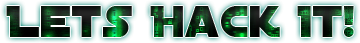# Encoding the sync word

First thing to do for frame sync is to convolution encode our sync word (0x1ACFFC1D). The LRIT signal uses a k=7

and r=1/2 with the poly’s 0x4F and 0x6D. We can use the parity function from libfec. So here is the code I use for encoding the word: http://codepad.org/3FJ1tVIG

``````#include <stdio.h>
#include <stdlib.h>
#include <stdint.h>
#include <fec.h>

int main(int argc,char *argv[]) {
int polys = {0x4f, 0x6d};
char syncword[] = {0x1A, 0xCF, 0xFC, 0x1D};
char outputword;

uint64_t encodedWord = 0;

char buff;

unsigned int encstate;
int wordSize = 4;
int processedBytes = 0;
int encodedWordPosition = 63;
int outputwordPosition = 0;

char c;

encstate = 0;
while(processedBytes < wordSize){
c = syncword[processedBytes];
for(int i=7;i>=0;i--){
encstate = (encstate << 1) | ((c >> 7) & 1);
c <<= 1;
// Soft Encoded Word
outputword[outputwordPosition] = 0 - parity(encstate & polys);
outputword[outputwordPosition + 1] = 0 - parity(encstate & polys);
outputwordPosition += 2;

// Encoded Word
encodedWord |= (uint64_t)(1-parity(encstate & polys)) << (encodedWordPosition);
encodedWord |= (uint64_t)(1-parity(encstate & polys)) << (encodedWordPosition-1);
encodedWordPosition-=2;
}
processedBytes++;
}

printf("Encoded Word: 0x%016lx\n", encodedWord);
printf("Soft Encoded Word: \n");
for (int i=0; i<64; i++) {
printf("0x%x ", outputword[i] & 0xFF);
}

printf("\n");

return 0;
}
``````

You can compile with gcc using:

``````gcc encword.c -lfec -lm -o encword
``````

This should give you the encoded syncword as 0xfca2b63db00d9794. But since we can have a phase ambiguity of 180 degrees in BPSK, we should also search for the inverted word that is 0x035d49c24ff2686b. Just side note, these are actually the inverted syncwords that we will use for searching the frame start. The 0 degree is atually 0x035d49c24ff2686b and 180 degree is 0xfca2b63db00d9794. But since we’ll use a correlation filter search, we should use the inverted words to get maximum correlation.

Also the program will output the Soft Symbols for that Encoded Sync Word, that is basically for every bit 0 it will have a byte 0, for every bit 1 it will have a byte 0xFF (256). That’s because we are sure that these are the correct bits (so we get the maximum values). In our decoder we’ll convert the uint64_t encoded syncwords to a soft symbol array to use in the correlation filter.